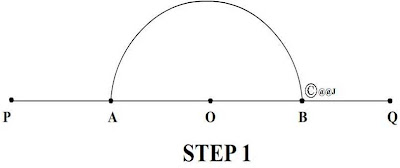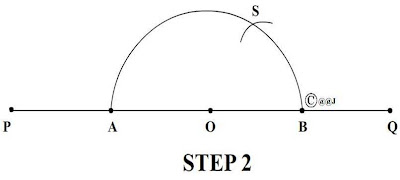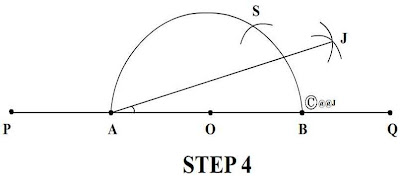# how can we draw a 50 degree angle.........without using D ????????can ny one tell plz??????plz tell

Without compass, we can not draw the angles which are not multiple of 15°.

Thus, it is not possible to draw the angle of 50° without compass.

• 20

CONSTRUCTING A 20 DEGREE ANGLE USING COMPASS AND STRAIGHTEDGE (RULER) ONLY

1. Step 1:
Draw a line segment PQ. With centre O, Draw an arc of any length using your compass and label it as AB.

2. Step 2:
Keeping your compass wide open with the same length, draw an arc S on AB. This will be your normal 60 Degree angle arc. (If you join S to O, you will get an angle measuring 60 degrees i.e. Angle SOB).

3. Step 3:
Bisect the 60 degree angle and name the point of intersection as J. J will be your normal 30 degree arc. (After bisecting the 60 degree angle, if you join J to O you will get an angle measuring 30 degrees i.e. Angle JOB).

Till Step 3, it was a normal construction. Step 4 is where the 'Twist' occurs!!!

4. Step 4:
Join the arc J to A, and there you have it! Angle JAB is your 20 degree angle! Now you can easily construct angles measuring 10, 40, 50, 70, 80, 100, 110, 130, 140, 160, 170 degrees!Now, construct 60 degree angle with AJ as the base. Now bisect that angle. You get 20 degree + 30 degree = 50 degree.

• 41

please tell me how to do it with 110 and 85

• -25
What are you looking for?